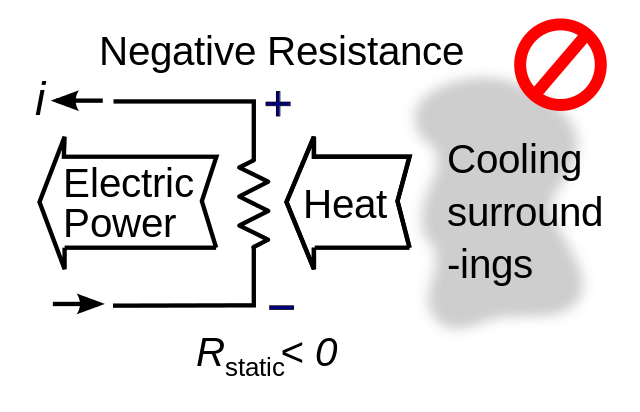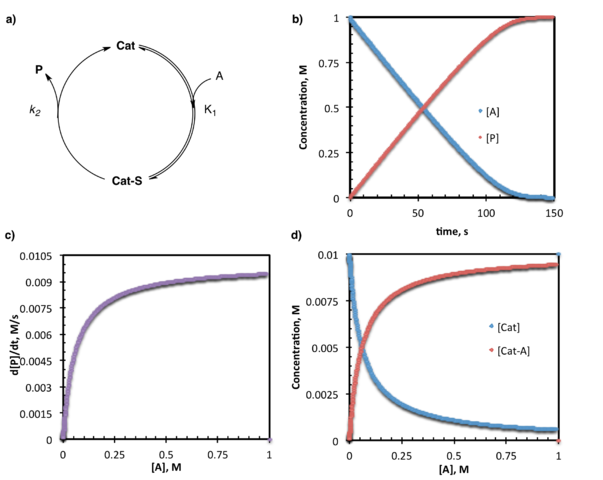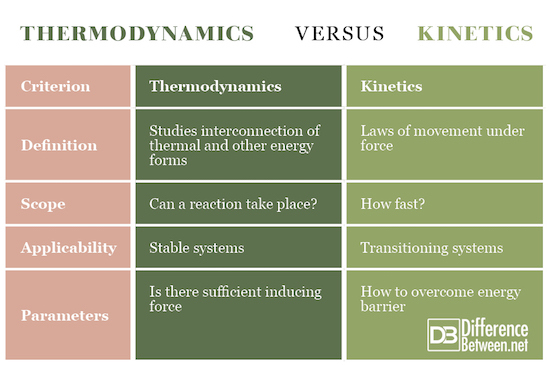#Difference Between Similar Terms and Objects

# Difference Between Thermodynamics and Kinetics

Similar to the molecular – kinetic theory of gases, thermodynamics is concerned with the analysis of gases. However, while the molecular-kinetic theory of gases studies gas processes with a micro approach, thermodynamics, on the other hand, has a macroscopic approach. This means that thermodynamics does not consider processes at a molecule level, but the gas is viewed as a whole, and gas processes are phenomenologically observed. The basic parameters are determined with observing the gas process.## What is Thermodynamics?

Thermodynamics is a science that studies the occurrences of mutual transformation of heat and other forms of energy (mechanical, chemical, electrical). It is a well-known fact that heat is received from doing a mechanical work.

We know that work transforms mechanical energy into heat. It is well known that heat can also be converted into mechanical work – for example heated water evaporates and that steam could trigger a turbine doing a mechanical work. However, this heat conversion is not simple and is never complete.

Resolving the relationship in transforming work into heat or heat into mechanical work has led to thermodynamics as a science.

Thermodynamics has evolved from the findings obtained through experiments and is based on experimentally determined laws, i.e. the laws of thermodynamics.

Let’s note: The first postulate equilibrium states that every system of natural bodies strives for a state of equilibrium, and when it achieves it, the system is no longer capable of changing itself on its own.

The second postulate equilibrium tells us that if one of two bodies in a heat balance is in balance with some third body, then the third body is also in balance with the remaining body. The second postulate of the equilibrium is also called the null law of thermodynamics. T

he first law of thermodynamics is the extension of the general natural law to the thermal phenomena. This is the law of conservation and conversion of energy. The second law of thermodynamics points to the course of the processes that take place in the nature that surrounds us and expresses the peculiarity of these processes. The third law of thermodynamics allows to uniquely determine the important thermodynamic size of the state – entropy.## What is Kinetics?

Kinetics is part of the theoretical mechanics that studies the movement of the bodies and the forces that cause this movement. Kinetics is a part of the mechanics that studies the position of the body and the material points (particles) describing its movement without analyzing the cause of the movement. To describe individual movements, it is also necessary to select the appropriate coordinate system on the basis of which the description of the movement will be carried out. One-dimensional (linear), two-dimensional (flat) and three-dimensional (spatial) coordinate systems are distinguished. On the other hand, kinetics is a part of mechanics that analyzes the motion of a rigid body/particle under the influence of force, describing the effect of force using different laws and relations. Problems solved by kinetics can be divided into two basic questions:

• How many forces act at the point if its movement is known? The solution to this question stems directly from Newton’s Law II, i.e. if the law of motion of a metrical point is known, the forces that produce this movement should be determined.
• What is the movement of a point if the known forces acting on a point are? This task is solved by integrating the differential equations of motion, i.e. If known forces acting on a metric point, the point movement is determined by integrating the differential equations of motion. In the technique, we mainly solve this second question, which is also called the basic task of dynamics (kinetics).

The task of kinetics is setting of differential equations of motion and their integration. Differential equations of motion point materials are derived from the basic law of dynamics – II Newton’s law.

## Difference Between Thermodynamics and Kinetics

1. ### Definition

Thermodynamics is a science that studies the phenomena created by the interconnection of thermal and other forms of energy (mechanical, chemical, electrical). Kinetics is a part of the theoretical mechanics in which the laws of the movement of the metrical bodies under the influence of force are studied. Kinetics studies the relationships between body movements and ICT-derived samples, i.e. strength and momentum.

1. ### Scope

Thermodynamics is concerned with whether a process (reaction) can take place, while kinetics analyzes the speed (rate) of the process.

1. ### Applicability

Thermodynamics is used in stable equilibrium systems, while kinetics is usable in transitioning systems.

1. ### Parameters

Thermodynamics shows if there is sufficient force present to induce a transformation. Kinetics shows how to overcome the energy barrier in order the complete transformation to be performed.

### Thermodynamics vs. Kinetics## Summary of Thermodynamics vs. Kinetics

• Thermodynamics is a science for heat and heat processes. It is built on the experience that man acquired on macroscopic bodies with common dimensions, with moderate density at moderate temperatures. It is a branch of physics that studies energy, its conversion between different forms, such as heat, and the ability to perform work. Thermodynamics deals with macroscopic systems with very high degrees of freedom.
• Kinetics is a part of dynamics that studies the effect of force on the movement of the body. It covers straight-line, circular motion, along the curve (e.g., parabolic motion). The principles of kinetics are Newton’s laws of motion, and D’Alembert’s principle of kinetic equilibrium or the same laws that are in line with the theory of relativity (in the region of high velocities, masses).

### Search DifferenceBetween.net :

Custom Search

Help us improve. Rate this post!Loading...Email This Post : If you like this article or our site. Please spread the word. Share it with your friends/family.

Please note: comment moderation is enabled and may delay your comment. There is no need to resubmit your comment.

## References :